Math Help: Memorize These Formulas

09/21/2015

Math Formulas to Know by Heart

Triangle Formulas

Area=1/2(b*h) Think of a triangle as half of a rectangle, so you need to take half of the base time height. The base of the triangle is the length of a side. If you have a right triangle, the height is the length of the side with the 90 degree angle. If non-right triangle, drop a line from the apex of the triangle to the base. Isosceles triangles have two equal sides and two equal angles. If one of the angles is 90 degrees, the other two will be 45. The lengths of a right isosceles triangle are always x, x, x√2. The find the hypotenuse, simply multiply √2 by one of the sides. The side lengths of a right triangle with angles 30-60-90 can be found using the formula x, x√3, and 2x. The smallest side, x, is opposite the smallest angle, 30. The longest side, 2x, is opposite the largest angle, 90.

Distance Formula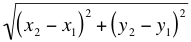If you know your Pythagorean theorem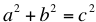, this equation will be intuitive. To determine the distance between two points, you can make a right triangle from them. square each side and then take the square root of the sum to obtain the length of the hypotenuse.

Logarithms

You should understand logarithms and how to rewrite them.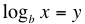asks b to what power (y) will result in x. You can rewrite it as: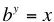. When x is a product of two different numbers, it may be easier to solve if you remember the following formula: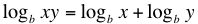Slope-Intercept Formula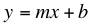In this formula, m is the slope and b is the y-intercept. If you get an equation that is not in this format, you will want to re-write it so that it is. It makes graphing a line much easier.

For Tutoring in Lake Forest, CA

When you need more help with math, contact The Tutoring Center in Lake Forest. We have one-to-one tutoring programs in math, reading, writing, and test prep. Find out more from our webpage and call 949 273 3534 to schedule a free diagnostic assessment.

FREE CONSULTATION

Schedule your Free Diagnostic Assessment Today!